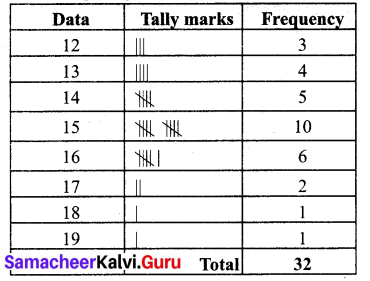Students can Download Maths Chapter 5 Statistics Ex 5.2 Questions and Answers, Notes Pdf, Samacheer Kalvi 7th Maths Book Solutions Guide Pdf helps you to revise the complete Tamilnadu State Board New Syllabus and score more marks in your examinations.

Tamilnadu Samacheer Kalvi 7th Maths Solutions Term 3 Chapter 5 Statistics Ex 5.2

Question 1.
Find the mode of the following data. 2, 4, 5, 2, 6, 7, 2, 7, 5,4, 8, 6, 10, 3, 2, 4, 2.
Solution:
Arranging the given data in ascending order 2, 2, 2, 2, 2, 3, 4, 4, 4, 5, 5, 6, 6, 7, 7, 8, 10.
Here the number 2 occurs 5 times which is the maximum
∴ Mode of this data is 2.

Question 2.
The number of points scored by a Kabaddi team in 20 matches are
36, 35, 27, 28, 29, 31, 32, 31, 35, 38, 38, 31, 28, 31, 34, 33, 34, 31, 30, 29.
Find the mode of the points scored by the team.
Solution:
Arranging the given data in ascending order
27, 28, 28, 29, 29, 30, 31, 31, 31, 31, 31, 32, 33, 34, 34, 35, 35, 36, 38, 38.
Here the number 31 occurs 5 times which is the maximum.
∴ Mode of this data is 31.Question 3.
The ages (in years) of 11 cricket players are given below.
25, 36, 39,38 40, 36, 25, 25, 38, o 26,36. Find the mode of the ages.
Solution:
Arranging the ages is ascending order: 25, 25, 25, 26, 36, 36, 36, 36, 38, 38, 39, 40.
The ages (in years) of 11 cricket players are given below. 25, 36, 39,38 40, 36, 25, 25, 38, o 26,36. Find the mode of the ages.
Arranging the ages is ascending order: 25, 25, 25, 26, 36, 36, 36, 36, 38, 38, 39, 40.
25 and 36 occurs maximum number of times.
∴ Mode is 25 and 36.

Question 4.
Find the mode of the following data.
12, 14, 12, 16, 15, 13, 14, 18, 19, 12, 14, 15, 16, 15, 16, 16, 15, 17, 13, 16, 16, 15, 15, 13, 15, 17, 15, 14, 15, 13, 15, 14.
Solution:
Tabulating the given dataThe highest frequency is 10 which corresponds to the value 15.
Hence mode of this data is 15.Objective Type Questions

Question 1.
The colors used by the six students for drawing is blue, orange, yellow, white, green and blue then the mode is ________
(i) blue
(ii) green
(iii) white
(iv) yellow
(i) blue

Question 2.
Find the mode of data 3, 6, 9, 12, 15.
(i) 1
(ii) 2
(iii) 3
(iv) No mode